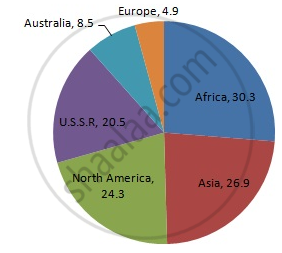Share

# Draw a Pie-diagram of the Areas of Continents of the World Given in the Following Table: - Mathematics

Course
ConceptCircle Graph Or Pie Chart - Drawing Pie Charts

#### Question

Draw a pie-diagram of the areas of continents of the world given in the following table:

 Continents Asia U.S.S.R Africa Europe Noth America South America Australia Area(in million sq. km) 26.9 20.5 30.3 4.9 24.3 17.9 8.5

#### Solution

We know:
Central angle of a component = (component value/sum of component values x 360)
Here, total area in million sq km = 133.3
Thus, the central angle for each component can be calculated as follows:

 Continent Area (in million sq. km) Sector angle Asia 26.9 26.9/133.3 x 360 = 72.6 U.S.S.R 20.5 20.5/133.3 x 360 = 55.4 Africa 30.3 30.3/133.3 x 360 = 81.8 Europe 4.9 4.9/133.3 x 360 = 13.2 North America 24.3 24.3/133.3 x 360 = 65.6 South America 17.9 17.9/133.3 x 360 = 48.3 Australia 8.5 8.5/133.3 x 360 = 23

Now, the pie chat representing the given data can be constructed by following the steps below:
Step 1 : Draw circle of an appropriate radius.
Step 2 : Draw a vertical radius of the circle drawn in step 1.
Step 3 : Choose the largest central angle. Here the largest central angle is 81.8o. Draw a sector with the central angle 81.8o in such a way that one of its radii coincides with the radius drawn in step 2 and another radius is in its counter clockwise direction.
Step 4 : Construct other sectors representing other items in the clockwise sense in descending order of magnitudes of their central angles.
​Step 5 : Shade the sectors with different colours and label them, as shown as in figure below.​​Is there an error in this question or solution?

#### APPEARS IN

RD Sharma Solution for Mathematics for Class 8 by R D Sharma (2019-2020 Session) (2017 to Current)
Chapter 25: Data Handling-III (Pictorial Representation of Data as Pie Charts or Circle Graphs)
Ex. 25.1 | Q: 9 | Page no. 13

#### Video TutorialsVIEW ALL 

Solution Draw a Pie-diagram of the Areas of Continents of the World Given in the Following Table: Concept: Circle Graph Or Pie Chart - Drawing Pie Charts.
S